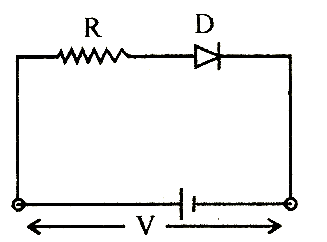# A d. c. battery of V volt is connected to a series combination of a resistor R and an ideal diode D as shown in the figure below. The potential difference across R will be(a)    2V when diode is forward biased (b)    Zero when diode is forward biased (c)    V when diode is reverse biased (d)    V when diode is forward biased

8 years ago

(b)

In forward biasing, the diode conducts. For ideal junction diode, the forward resistance is zero; therefore, entire applied voltage occurs across external resistance R i.e., there occurs no potential drop, so potential across R is V in forward biased.